# How to write exponentiation and exponential functions in LaTeX?

The use of superscripts has been shown in each case of this content. Exponentiation is formed by the combination of base and exponent or power.

(base)(exp) like xn, ak.

Power or exponent will always be defined by superscript, for which latex has base^{exponent} syntax.

\documentclass{article}
\begin{document}
$(Base)^{exponent}$
$x^n,K^n,p^q$
$e^{\ln(x)}$
\end{document}

Output :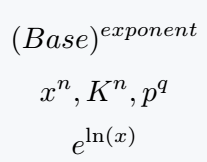If the length of the exponent or power is more than a single character, the whole exponent must pass through the curly bracket.

\documentclass{article}
\begin{document}
$x^pq \quad x^{pq}$
$c^nk \quad c^{nk}$
$x^a\ln (x) \quad x^{a\ln (x)}$
\end{document}

Output :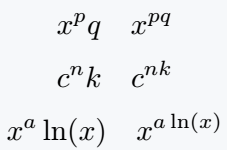## Exponential functions in LaTeX

Exponential functions are written in two ways. The first uses the base as e and the second uses the exponent as an argument in the exp function.

In latex, There is a pre-defined command for exp function.

\documentclass{article}
\begin{document}
$e^x \quad \exp(x)$
$e^{\ln(x)} \quad \exp(\ln x)$
$e^{n^2} \quad \exp(n^2)$
$e^{1/x} \quad \exp(1/x)$
$e^{\ln x/x} \quad \exp^{\ln x/x}$
$(e^z)^n \quad \exp(nz)$
\end{document}

Output :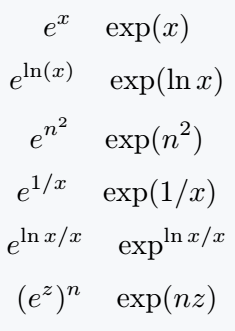## epow and exp(pow) which is best?

Have you ever wondered why this exponential function is defined in two ways? The main reason for this is to maintain the beauty of mathematical expression.

Below are some examples that will help you differentiate between the two cases.

And you can easily understand what is right and what is wrong.

\documentclass{article}
\begin{document}
$e^{(\frac{x}{x+1})} \quad \exp\left(\frac{x}{x+1}\right)$
$e^{\ln x +k} \quad \exp(\ln x + k)$
$e^{(\frac{\sin x}{x})} \quad \exp\left(\frac{\sin x}{x}\right)$
$e^{\ln x^{\ln x}} \exp\left(\ln x^{\ln x}\right)$
\end{document}

Output :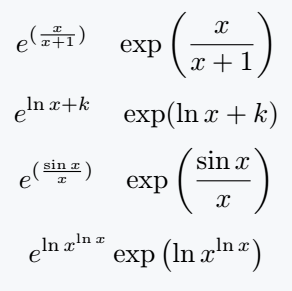## Use continuous fractions for ex

You may have noticed that the exponential function is written with the help of continuous fraction.

But in this case,  \cfrac{num}{den} best practice instead of \frac{num}{den} command.

\documentclass{article}
\usepackage{amsmath}
\begin{document}
$e^x = 1+\cfrac{x}{1-\cfrac{x}{x+2 -\cfrac{2x}{x+3-\cfrac{3x}{x+4-\cfrac{4x}{\ddots}}}}}$
$e^z = 1+\cfrac{2z}{2-z+\cfrac{z^2}{6 +\cfrac{z^2}{10+\cfrac{z^2}{14+\cfrac{z^2}{\ddots}}}}}$
\end{document}

Output :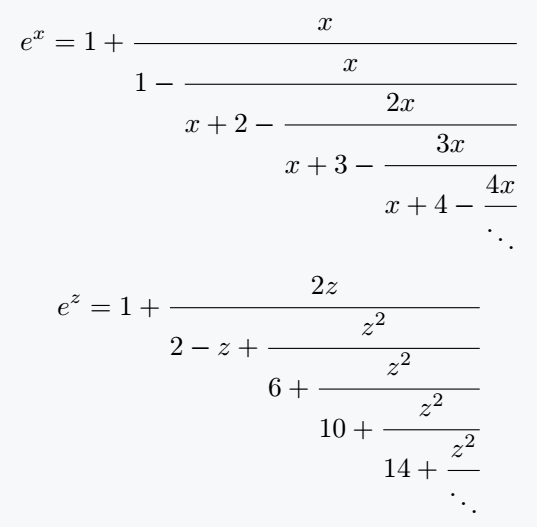#### Md Jidan Mondal

LaTeX expert with over 10 years of experience in document preparation and typesetting. Specializes in creating professional documents, reports, and presentations using LaTeX.